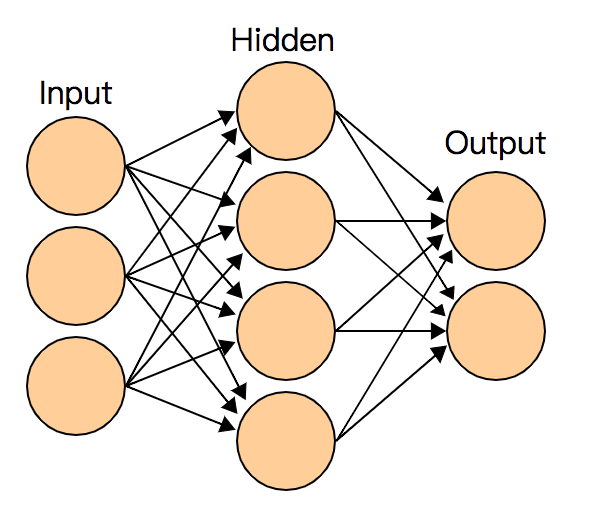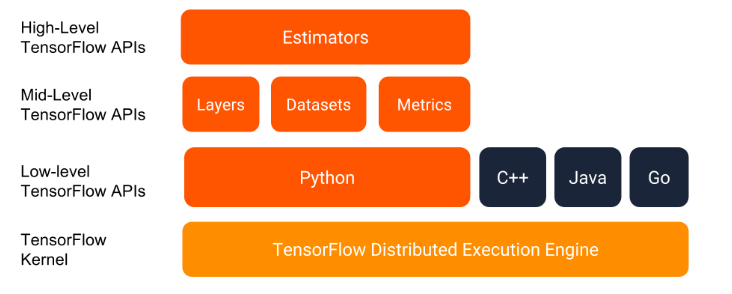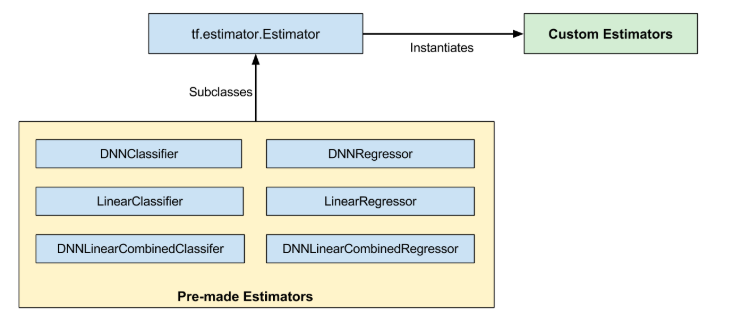# Community

Blog Events Webinars Tutorials Forum
×
Community Blog DNN training for LibSVM-formatted data - From Keras to Estimator

# DNN training for LibSVM-formatted data - From Keras to Estimator

This article provides a fully verified solution (with code) to run LR and GBDT on a LibSVM-formatted dataset efficiently using TensorFlow.

## Background

Suppose you have a LibSVM-formatted dataset that has run LR and GBDT, and want to know the effect of DNN quickly. Then, this article is for you.

Although the popularity of deep learning research and application has been on the rise for many years, and TensorFlow is well known amongst users who specialize in algorithms, not everyone is familiar with this tool. In addition, it is not a matter of instantly building a simple DNN model based on a personal dataset, especially when the dataset is in LibSVM format. LibSVM is a common format for machine learning, and is supported by many tools, including Liblinear, XGBoost, LightGBM, ytk-learn and xlearn. However, TensorFlow has not provided an elegant solution for this either officially or privately, which has caused a lot of inconvenience for new users, and is unfortunate considering it is such a widely used tool. To this end, this article provides a fully verified solution (some code), which I believe can help new users save some time.

## Introduction

The code in this article can be used:

• To quickly verify the effect of the LibSVM-formatted dataset on the DNN model, to compare it with other linear models or tree models and explore the limits of the model.
• To reduce the dimension of high-dimensional features. The output of the first hidden layer can be used as the Embedding, and added to other training processes.
• To get started with TensorFlow Keras, Estimator, and Dataset for beginners.

The coding follows the following principles:

• Instead of developing your own code, you should try to use official or other code that is recognized as having the best performance unless you have to.
• The code should be as streamlined as possible.
• The ultimate time and space complexity is pursued.

This article only introduces the most basic DNN multi-class classification training evaluation code. For more advanced complex models, see outstanding open-source projects, such as DeepCTR. Subsequent articles will share the application of these complex models in practical research.Below are four advanced code and concepts for TensorFlow to train DNN for LibSVM-formatted data. The latter two are recommended.

## Keras Generator

Here are three choices:

• TensorFlow API: Building a DNN model using TensorFlow is easy for skilled users. A DNN model can be built right away with a low-level API, but the code is a slightly messy. By contrast, the high-level API Keras is more "considerate", and the code is extremely streamlined and clear at a glance.
• LibSVM-formatted data reading: It is easy to write the code that reads data in LibSVM format. You can simply convert the sparse coding into dense coding. However, since sklearn has already provided load_svmlight_file, why not use it? This function will read the entire file into the memory, which is feasible for smaller data sizes.
• fit and fit_generator: Keras model training only receives dense coding, while LibSVM uses sparse coding. If the dataset is not too large, it can be read into the memory through load_svmlight_file. However, if you convert all the data into dense coding and then feed it to fit, the memory may crash. The ideal solution is to read the data into the memory on demand, and then convert it. Here, for convenience, all the data is read into the memory using load_svmlight_file, and saved in sparse coding. And, it is then fed to fit_generator in batches during use.

The code is as follows:

``````import numpy as np
from sklearn.datasets import load_svmlight_file
from tensorflow import keras
import tensorflow as tf

feature_len = 100000 # 特征维度，下面使用时可替换成 X_train.shape
n_epochs = 1
batch_size = 256
train_file_path = './data/train_libsvm.txt'
test_file_path = './data/test_libsvm.txt'

def batch_generator(X_data, y_data, batch_size):
number_of_batches = X_data.shape/batch_size
counter=0
index = np.arange(np.shape(y_data))
while True:
index_batch = index[batch_size*counter:batch_size*(counter+1)]
X_batch = X_data[index_batch,:].todense()
y_batch = y_data[index_batch]
counter += 1
yield np.array(X_batch),y_batch
if (counter > number_of_batches):
counter=0

def create_keras_model(feature_len):
model = keras.Sequential([
# 可在此添加隐层
keras.layers.Dense(64, input_shape=[feature_len], activation=tf.nn.tanh),
keras.layers.Dense(6, activation=tf.nn.softmax)
])
loss='sparse_categorical_crossentropy',
metrics=['accuracy'])
return model

if __name__ == "__main__":
X_train, y_train = load_svmlight_file(train_file_path)
X_test, y_test = load_svmlight_file(test_file_path)

keras_model = create_keras_model(X_train.shape)

keras_model.fit_generator(generator=batch_generator(X_train, y_train, batch_size = batch_size),
steps_per_epoch=int(X_train.shape/batch_size),
epochs=n_epochs)

test_loss, test_acc = keras_model.evaluate_generator(generator=batch_generator(X_test, y_test, batch_size = batch_size),
steps=int(X_test.shape/batch_size))
print('Test accuracy:', test_acc)``````

The code above is the code used in practical research earlier. It was able to complete the training task at that time, but the shortcomings of the code are obvious. On the one hand, the space complexity is low. The resident memory of big data will affect other processes. When it comes to big datasets, it is ineffective. On the other hand, the availability is poor. The batch_generator needs to be manually encoded to realize the batch of data, which is time-consuming and error-prone.

TensorFlow Dataset is a perfect solution. However, I was not familiar with Dataset and did not know how to use TF low-level API to parse LibSVM and convert SparseTensor to DenseTensor, so I put it on hold due to limited time at that time, and the problem was solved later. The key point is the decode_libsvm function in the following code.

After LibSVM-formatted data is converted into Dataset, DNN is unlocked and can run freely on any large dataset.

The following in turn describes the application of Dataset in Keras model, Keras to Estimator, and DNNClassifier.

The following is the Embedding code. The output of the first hidden layer is used as Embedding:

``````def save_output_file(output_array, filename):
result = list()
for row_data in output_array:
line = ','.join([str(x) for x in row_data.tolist()])
result.append(line)
with open(filename,'w') as fw:
fw.write('%s' % '\n'.join(result))

X_test, y_test = load_svmlight_file("./data/test_libsvm.txt")
dense1_layer_model = Model(inputs=model.input, outputs=model.layers.output)
dense1_output = dense1_layer_model.predict(X_test)
save_output_file(dense1_output, './hidden_output/hidden_output_test.txt')``````

## Keras Dataset

The LibSVM-formatted data read by load_svmlight_file is changed to Dataset read by decode_libsvm.

``````import numpy as np
from sklearn.datasets import load_svmlight_file
from tensorflow import keras
import tensorflow as tf

feature_len = 138830
n_epochs = 1
batch_size = 256
train_file_path = './data/train_libsvm.txt'
test_file_path = './data/test_libsvm.txt'

def decode_libsvm(line):
columns = tf.string_split([line], ' ')
labels = tf.string_to_number(columns.values, out_type=tf.int32)
labels = tf.reshape(labels,[-1])
splits = tf.string_split(columns.values[1:], ':')
id_vals = tf.reshape(splits.values,splits.dense_shape)
feat_ids, feat_vals = tf.split(id_vals,num_or_size_splits=2,axis=1)
feat_ids = tf.string_to_number(feat_ids, out_type=tf.int64)
feat_vals = tf.string_to_number(feat_vals, out_type=tf.float32)
# 由于 libsvm 特征编码从 1 开始，这里需要将 feat_ids 减 1
sparse_feature = tf.SparseTensor(feat_ids-1, tf.reshape(feat_vals,[-1]), [feature_len])
dense_feature = tf.sparse.to_dense(sparse_feature)
return dense_feature, labels

def create_keras_model():
model = keras.Sequential([
keras.layers.Dense(64, input_shape=[feature_len], activation=tf.nn.tanh),
keras.layers.Dense(6, activation=tf.nn.softmax)
])
loss='sparse_categorical_crossentropy',
metrics=['accuracy'])
return model

if __name__ == "__main__":
dataset_train = tf.data.TextLineDataset([train_file_path]).map(decode_libsvm).batch(batch_size).repeat()
dataset_test = tf.data.TextLineDataset([test_file_path]).map(decode_libsvm).batch(batch_size).repeat()

keras_model = create_keras_model()

sample_size = 10000 # 由于训练函数必须要指定 steps_per_epoch，所以这里需要先获取到样本数
keras_model.fit(dataset_train, steps_per_epoch=int(sample_size/batch_size), epochs=n_epochs)

test_loss, test_acc = keras_model.evaluate(dataset_test, steps=int(sample_size/batch_size))
print('Test accuracy:', test_acc)``````

This solves the problem with high space complexity, and ensures the data does not occupy a large amount of memory.

However, in terms of availability, two inconveniences still exist:

• When the Keras "fit" function is used, steps_per_epoch must be specified. To ensure that the entire batch of data is completed in each round, the sample size must be computed in advance, which is unreasonable. In fact, the dataset.repeat can ensure the entire batch of data is completed in each round. If Estimator is used, it is not necessary to specify steps_per_epoch.
• The feature dimension feature_len needs to be computed in advance. LibSVM uses sparse coding, so it is impossible to infer the feature dimension by reading only one or a few rows of data. You can use load_svmlight_file offline to obtain the feature dimension feature_len=X_train.shape, and then hard code it into the code. This is an inherent feature of LibSVM. Therefore, this is the only way to deal with it.

## Keras Model to Estimator

Another high-level API of TensorFlow is Estimator, which is more flexible. Its standalone code is consistent with the distributed code, and the underlying hardware facilities need not be considered, so it can be conveniently combined with some distributed scheduling frameworks (such as xlearning). In addition, Estimator seems to gets more comprehensive support from TensorFlow than Keras.Estimator is a high-level API that is independent from Keras. If Keras was used before, it is impossible to reconstruct all the data into Estimator in a short time. TensorFlow also provides the model_to_estimator interface for Keras models, which can also benefit from Estimator.

``````from tensorflow import keras
import tensorflow as tf
from tensorflow.python.platform import tf_logging
# 打开 estimator 日志，可在训练时输出日志，了解进度
tf_logging.set_verbosity('INFO')

feature_len = 100000
n_epochs = 1
batch_size = 256
train_file_path = './data/train_libsvm.txt'
test_file_path = './data/test_libsvm.txt'

# 注意这里多了个参数 input_name，返回值也与上不同
def decode_libsvm(line, input_name):
columns = tf.string_split([line], ' ')
labels = tf.string_to_number(columns.values, out_type=tf.int32)
labels = tf.reshape(labels,[-1])
splits = tf.string_split(columns.values[1:], ':')
id_vals = tf.reshape(splits.values,splits.dense_shape)
feat_ids, feat_vals = tf.split(id_vals,num_or_size_splits=2,axis=1)
feat_ids = tf.string_to_number(feat_ids, out_type=tf.int64)
feat_vals = tf.string_to_number(feat_vals, out_type=tf.float32)
sparse_feature = tf.SparseTensor(feat_ids-1, tf.reshape(feat_vals,[-1]),[feature_len])
dense_feature = tf.sparse.to_dense(sparse_feature)
return {input_name: dense_feature}, labels

def input_train(input_name):
# 这里使用 lambda 来给 map 中的 decode_libsvm 函数添加除 line 之的参数
return tf.data.TextLineDataset([train_file_path]).map(lambda line: decode_libsvm(line, input_name)).batch(batch_size).repeat(n_epochs).make_one_shot_iterator().get_next()

def input_test(input_name):
return tf.data.TextLineDataset([train_file_path]).map(lambda line: decode_libsvm(line, input_name)).batch(batch_size).make_one_shot_iterator().get_next()

def create_keras_model(feature_len):
model = keras.Sequential([
# 可在此添加隐层
keras.layers.Dense(64, input_shape=[feature_len], activation=tf.nn.tanh),
keras.layers.Dense(6, activation=tf.nn.softmax)
])
loss='sparse_categorical_crossentropy',
metrics=['accuracy'])
return model

def create_keras_estimator():
model = create_keras_model()
input_name = model.input_names
estimator = tf.keras.estimator.model_to_estimator(model)
return estimator, input_name

if __name__ == "__main__":
keras_estimator, input_name = create_keras_estimator(feature_len)
keras_estimator.train(input_fn=lambda:input_train(input_name))
eval_result = keras_estimator.evaluate(input_fn=lambda:input_train(input_name))
print(eval_result)``````

Here, sample_size need not be computed, but feature_len still needs to be computed in advance. Note that the "dict key" returned by input_fn of Estimator must be consistent with the input name of the model. This value is passed through input_name.

Many people use Keras, and Keras is also used in many open-source projects to build complex models. Due to the special format of Keras models, they cannot be saved in some platforms, but these platforms support Estimator models to be saved. In this case, it is very convenient to use model_to_estimator to save Keras models.

## DNNClassifier

Finally, let's directly use the Estimator pre-created by TensorFlow: DNNClassifier.``````import tensorflow as tf
from tensorflow.python.platform import tf_logging
# 打开 estimator 日志，可在训练时输出日志，了解进度
tf_logging.set_verbosity('INFO')

feature_len = 100000
n_epochs = 1
batch_size = 256
train_file_path = './data/train_libsvm.txt'
test_file_path = './data/test_libsvm.txt'

def decode_libsvm(line, input_name):
columns = tf.string_split([line], ' ')
labels = tf.string_to_number(columns.values, out_type=tf.int32)
labels = tf.reshape(labels,[-1])
splits = tf.string_split(columns.values[1:], ':')
id_vals = tf.reshape(splits.values,splits.dense_shape)
feat_ids, feat_vals = tf.split(id_vals,num_or_size_splits=2,axis=1)
feat_ids = tf.string_to_number(feat_ids, out_type=tf.int64)
feat_vals = tf.string_to_number(feat_vals, out_type=tf.float32)
sparse_feature = tf.SparseTensor(feat_ids-1,tf.reshape(feat_vals,[-1]),[feature_len])
dense_feature = tf.sparse.to_dense(sparse_feature)
return {input_name: dense_feature}, labels

def input_train(input_name):
return tf.data.TextLineDataset([train_file_path]).map(lambda line: decode_libsvm(line, input_name)).batch(batch_size).repeat(n_epochs).make_one_shot_iterator().get_next()

def input_test(input_name):
return tf.data.TextLineDataset([train_file_path]).map(lambda line: decode_libsvm(line, input_name)).batch(batch_size).make_one_shot_iterator().get_next()

def create_dnn_estimator():
input_name = "dense_input"
feature_columns = tf.feature_column.numeric_column(input_name, shape=[feature_len])
estimator = tf.estimator.DNNClassifier(hidden_units=,
n_classes=6,
feature_columns=[feature_columns])
return estimator, input_name

if __name__ == "__main__":
dnn_estimator, input_name = create_dnn_estimator()
dnn_estimator.train(input_fn=lambda:input_train(input_name))

eval_result = dnn_estimator.evaluate(input_fn=lambda:input_test(input_name))
print('\nTest set accuracy: {accuracy:0.3f}\n'.format(**eval_result))``````

The logic of Estimator code is clear, and it is easy to use, and very powerful. For more information about Estimator, see official documentation.

The above solutions, except for the first one, which doesn't conveniently process big data, can be run on a single machine, and the network structure and target functions can be modified as needed when in use.

The code in this article is derived from a survey, which takes several hours to debug. The code is "idle" after the survey is completed. This code is now shared for reference, and I hope it can be helpful to other users.

0 0 0
Share on

# Related Products

• ##DataWorks

A secure environment for offline data development, with powerful Open APIs, to create an ecosystem for redevelopment.

• ##MaxCompute

Conduct large-scale data warehousing with MaxCompute

• ##Machine Learning Platform For AI

An end-to-end platform that provides various machine learning algorithms to meet your data mining and analysis requirements.